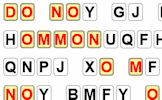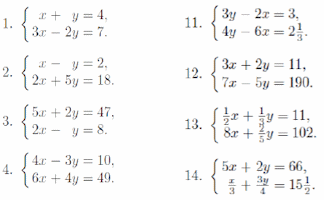# Simultaneous Equations

## A self-marking, multi-level set of exercises on solving pairs of simultaneous equations.

##### MenuLevel 1Level 2Level 3Level 4Level 5Level 6Level 7ExamHelpMore

This is level 1: equations that can be added or subtracted to eliminate one variable. You will be awarded a trophy if you get at least 9 correct and you do this activity online.

 3x + y = 322x + y = 24 x= y=2x + y = 25x + y = 17 x= y=3a + 4b = 333a + 2b = 27 a= b=2c + 2d = 164c + 2d = 22 c= d=-e + 5f = 26-e − 3f = -22 e= f=3g + 5h = 9-2g − 5h = -1 g= h=j + 3k = -32-j + 4k = -31 j= k=-4m + 2n = -10-4m − 2n = 26 m= n=-6p + 10q = -26p − 3q = -12 p= q=-4r + 7s = -466r − 7s = 62 r= s=-12u − 6v = 18-12u − 11v = 53 u= v=6w + 6z = 6-6w + 6z = 54 w= z=Check

This is Simultaneous Equations level 1. You can also try:
Level 2 Level 3 Level 4 Level 5 Level 6 Level 7

## Instructions

Try your best to answer the questions above. Type your answers into the boxes provided leaving no spaces. As you work through the exercise regularly click the "check" button. If you have any wrong answers, do your best to do corrections but if there is anything you don't understand, please ask your teacher for help.

When you have got all of the questions correct you may want to print out this page and paste it into your exercise book. If you keep your work in an ePortfolio you could take a screen shot of your answers and paste that into your Maths file.

## Transum.org

This web site contains over a thousand free mathematical activities for teachers and pupils. Click here to go to the main page which links to all of the resources available.## More Activities:

Mathematicians are not the people who find Maths easy; they are the people who enjoy how mystifying, puzzling and hard it is. Are you a mathematician?

Comment recorded on the 19 November 'Starter of the Day' page by Lesley Sewell, Ysgol Aberconwy, Wales:

"A Maths colleague introduced me to your web site and I love to use it. The questions are so varied I can use them with all of my classes, I even let year 13 have a go at some of them. I like being able to access Starters for the whole month so I can use favourites with classes I see at different times of the week. Thanks."

Comment recorded on the 14 September 'Starter of the Day' page by Trish Bailey, Kingstone School:

"This is a great memory aid which could be used for formulae or key facts etc - in any subject area. The PICTURE is such an aid to remembering where each number or group of numbers is - my pupils love it!
Thanks"

Each month a newsletter is published containing details of the new additions to the Transum website and a new puzzle of the month.

The newsletter is then duplicated as a podcast which is available on the major delivery networks. You can listen to the podcast while you are commuting, exercising or relaxing.

Transum breaking news is available on Twitter @Transum and if that's not enough there is also a Transum Facebook page.

#### Code CrackerLearn the basic techniques for cracking codes then practise them using this interactive challenge. There are three levels of difficulty and many different messages to decipher.

There are answers to this exercise but they are available in this space to teachers, tutors and parents who have logged in to their Transum subscription on this computer.

A Transum subscription unlocks the answers to the online exercises, quizzes and puzzles. It also provides the teacher with access to quality external links on each of the Transum Topic pages and the facility to add to the collection themselves.

Subscribers can manage class lists, lesson plans and assessment data in the Class Admin application and have access to reports of the Transum Trophies earned by class members.

Subscribe

## Go Maths

Learning and understanding Mathematics, at every level, requires learner engagement. Mathematics is not a spectator sport. Sometimes traditional teaching fails to actively involve students. One way to address the problem is through the use of interactive activities and this web site provides many of those. The Go Maths page is an alphabetical list of free activities designed for students in Secondary/High school.

## Maths Map

Are you looking for something specific? An exercise to supplement the topic you are studying at school at the moment perhaps. Navigate using our Maths Map to find exercises, puzzles and Maths lesson starters grouped by topic.

## Teachers

If you found this activity useful don't forget to record it in your scheme of work or learning management system. The short URL, ready to be copied and pasted, is as follows:

Alternatively, if you use Google Classroom, all you have to do is click on the green icon below in order to add this activity to one of your classes.

It may be worth remembering that if Transum.org should go offline for whatever reason, there are mirror sites at Transum.com and Transum.info that contain most of the resources that are available here on Transum.org.

When planning to use technology in your lesson always have a plan B!

Do you have any comments? It is always useful to receive feedback and helps make this free resource even more useful for those learning Mathematics anywhere in the world. Click here to enter your comments.For All:

Scan the QR code below to visit the online version of this activity.https://Transum.org/go/?Num=100

## Description of Levels

CloseProjectable - A set of simultaneous equations designed to be shown one at a time to the whole class.

Level 1 - Equations that can be added or subtracted to eliminate one variable.

Level 2 - Equations that can be added or subtracted to eliminate one variable after one of the equations has been multiplied by a constant.

Level 3 - Equations that can be added or subtracted to eliminate one variable after both of the equations have been multiplied by constants.

Level 4 - Equations with two variables that are not written in the standard way.

Level 5 - Real life problems that can be solved by writing them as simultaneous equations.

Level 6 - Equations which include fractions in some way.

Level 7 - Linear, quadratic and other pairs of simultaneous equations.

These Level 7 questions will require you to be able to solve Quadratic Equations.

Exam Style Questions - A collection of problems in the style of GCSE or IB/A-level exam paper questions (worked solutions are available for Transum subscribers).

More Simultaneous Equations including lesson Starters, visual aids, investigations and self-marking exercises.

## Extension

There is a printable worksheet to go with this activity. It is an exercise that appeared in an algebra book published in 1895. It starts with basic questions but soon gets tricky!Answers to this exercise are available lower down this page when you are logged in to your Transum account. If you don’t yet have a Transum subscription one can be very quickly set up if you are a teacher, tutor or parent.

## Curriculum Reference

See the National Curriculum page for links to related online activities and resources.

## Help Video

The examples used in the video are available to teachers as projectable slides.

## Level 1 Example

$$3x+y=34 \qquad \mathbf{A}\\2x+y=25 \qquad \mathbf{B}$$

You may notice that each equation has a $$y$$ term so subtracting one equation from the other would result in the elimination of that term.

Subtract $$\mathbf{B}$$ from $$\mathbf{A}$$

$$x=9$$

Substitute this value for $$x$$ into equation $$\mathbf{A}$$.

$$27+y=34$$
$$y=7$$

The simultaneous equations have been solved.
The solutions are $$x=9$$ and $$y=7$$.

You can check your answers by substituting them both into equation $$\mathbf{B}$$ to see if it balances.

This example is not intended to teach you everything you need to know about this type of simultaneous equations. It is here as a reminder and is no substitute for your teacher or tutor.

Don't wait until you have finished the exercise before you click on the 'Check' button. Click it often as you work through the questions to see if you are answering them correctly. You can double-click the 'Check' button to make it float at the bottom of your screen.

Answers to this exercise are available lower down this page when you are logged in to your Transum account. If you don’t yet have a Transum subscription one can be very quickly set up if you are a teacher, tutor or parent.

Close